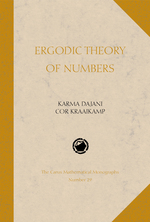# Ergodic Theory of Numbers

Karma Dajani
Cor Kraaikamp
Volume: 29
Edition: 1
Pages: 201
https://www.jstor.org/stable/10.4169/j.ctt5hh8rr

1. Front Matter
(pp. i-vi)
(pp. vii-viii)
3. Preface
(pp. ix-x)
Karma Dajani and Cor Kraaikamp
4. CHAPTER 1 Introduction
(pp. 1-32)

Letxbe a real number from the unit interval [0,1). As is well known,xcan be written as

$x = \sum\limits_{k = 1}^\infty {\frac{{{a_k}(x)}}{{{{10}^k}}}}$, (1.1)

where${a_k} = {a_k}(x) \in \left\{ {0,1, \ldots ,9} \right\}$for$k \ge 1$. We will denote (1.1) by$x = .{a_1}{a_2} \ldots {a_k} \ldots .$This expansion ofx, the so-calleddecimal expansion, is unique if we do not allow infinite expansions with$k \ge 1$from some${k_0}$on, or conversely, if we allow only infinite expansions. So for example we can write the (rational) number$x = {10^{ - 2}}$in the following two ways, as either .01 or .0099999 ...99999 ....

Decimal expansions have become such an integral part of our daily life that it takes...

5. CHAPTER 2 Variations on a theme (Other expansions)
(pp. 33-56)

In this chapter we investigate how far the dynamics of the decimal expansion can be generalized without losing its ‘essential properties’, like Bernoullicity. We will see that the underlying property that guarantees, for instance, independence of the digits is that the mapTgenerating the expansion is piecewise linear onto [0, 1) on each partition element.

The ideas and results for the decimal expansion go through in exactly the same way if we look atn-ary expansions of numbers in [0,1). Here,$n \ge 1$is an integer and every irrational number$x \in [0,1)$can be uniquely written

$x = \sum\limits_{k = 1}^\infty {\frac{{{a_k}(x)}}{{{n^k}}}}$,${a_k} = {a_k}(x) \in \left\{ {0,1, \ldots ,n - 1} \right\}$.

Exercise 2.1.1. Let...

6. CHAPTER 3 Ergodicity
(pp. 57-88)

Why is the existence of aT-invariant measure important? The reason is that it allows us to use ergodic theory to study the behavior of the mapT. In particular, it makes available the use of the Ergodic Theorem with which answers to many number-theoretical questions can be given (like frequency of digits).

The wordergodiccomes from the Greek (ergon= work andodos= path), and originates from physics. In the 1920s and 1930s it drew the attention of mathematicians. In this chapter we will present some of the basic results in ergodic theory.

Before we proceed we...

7. CHAPTER 4 Systems obtained from other systems
(pp. 89-114)

In this chapter we will show that there is a deep relationship between GLS expansion andβ-expansion. A similar relationship will be used in Chapter 5 to find many metric and Diophantine properties of the regular and (infinitely many) other continued fraction expansions.

Let us once more return to the golden mean example. Recall that$\beta = \frac{1}{2}(\sqrt 5 + 1)$satisfies${z^2} - z - 1 = 0$, and therefore we have

$1 = \frac{1}{\beta } + \frac{1}{{{\beta ^2}}}$;

see also Example 3.4.1. Now${T_\beta }$is given by

${T_\beta }x = \left\{ {\begin{array}{*{20}{c}} {\beta x} \\ {\beta x - 1} \\ \end{array}} \right.$,$x \in [0,\frac{1}{\beta })$,$x \in [\frac{1}{\beta },1)$.

Let${\rm T} = \left\{ {[0,\frac{1}{\beta }),[\frac{1}{\beta },1)} \right\}$and letSbe the GLS(T) transformation (see Section 2.3.1). Thus

$Sx = \left\{ {\begin{array}{*{20}{c}} {\beta x,} \\ {{\beta ^2}x - \beta ,} \\ \end{array}} \right.$$x \in [0,\frac{1}{\beta })$,$x \in [\frac{1}{\beta },1)$.

Notice that for$x \in [0,\frac{1}{\beta })$we have$Sx = {T_\beta }x$and for...

8. CHAPTER 5 Diophantine approximation and continued fractions
(pp. 115-154)

In Section 1.3.1 continued fractions were introduced as a generalization of Euclid’s algorithm. Although at first view they appear strange, continued fractions play an important role at many places in mathematics. For instance they appear inprimality testing, which is not so surprising, since Euclid’s algorithm is also a test whether two integersmandnare relatively prime. For more details, see the book by Bressoud [Bre89]. There is also an important relation between continued fractions andalgebraic geometry, see the nice introductory papers by C. Series [Ser82] and [Ser85].

Another important application of continued fractions is theapproximation...

9. CHAPTER 6 Entropy
(pp. 155-178)

Given a measure preserving transformationTon a probability space (X,F,µ) , we want to define a nonnegative quantityh(T) that measures the average uncertainty about whereTmoves the points ofX. That is, the value ofh(T) reflects the amount of randomness generated byT. We want to defineh(T) in such a way that (i) the amount of information gained by an application ofTis proportional to the amount of uncertainty removed, and (ii)h(T) is isomorphism invariant, so that isomorphic transformations have equal entropy.

The connection between entropy (that is...

10. Bibliography
(pp. 179-186)
11. Index
(pp. 187-190)